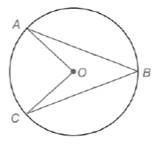Chapter 6.1, Problem 40E### Elementary Geometry for College St...

6th Edition
Daniel C. Alexander + 1 other
ISBN: 9781285195698

#### Solutions

Chapter
Section### Elementary Geometry for College St...

6th Edition
Daniel C. Alexander + 1 other
ISBN: 9781285195698
Textbook Problem
1 views

# Use a paragraph proof to complete this exercise. Given: ⊙ O with chords A B ¯ and B C ¯ , radii A O ¯ and O C ¯ Prove: m ∠ A B C < m ∠ A O CTo determine

To prove:

In a circle with centre O, AB¯ and BC¯ are the chords and AO¯,OC¯ are radii, then mABC<mAOC.

Explanation

Given:

In a circle with centre O, AB¯ and BC¯ are the chords and AO¯,OC¯ are radii.

Theorem used:

The measure of an inscribed angle of a circle is one-half the measure of its intercepted arc.

Central angle postulate:

In a circle, the degree measure of a central angle is equal to the degree measure of its intercepted arc.

Proof:

Consider a circle with center O.

Let AB¯ and BC¯ be the chords and let AO¯,OC¯ be radii of the circle.

From the figure, we see that AOC is the central angle.

By central angle postulate, mAOC=mAC.

From the figure we see that ABC is the inscribed angle with the intercepted arc AC

### Still sussing out bartleby?

Check out a sample textbook solution.

See a sample solution

#### The Solution to Your Study Problems

Bartleby provides explanations to thousands of textbook problems written by our experts, many with advanced degrees!

Get Started

#### Find the derivatives of the functions in Problems 1-34. 10.

Mathematical Applications for the Management, Life, and Social Sciences

#### True or False: is a convergent series.

Study Guide for Stewart's Multivariable Calculus, 8th

#### For f(x)=xx+1,f(x)=. a) 1 b) x2(x+1)2 c) 1(x+1)2 d) 1(x+1)2

Study Guide for Stewart's Single Variable Calculus: Early Transcendentals, 8th